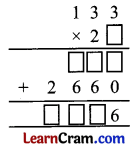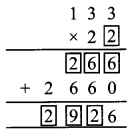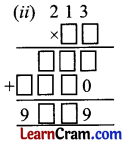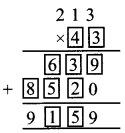# DAV Class 4 Maths Chapter 3 Worksheet 4 Solutions

The DAV Class 4 Maths Book Solutions and DAV Class 4 Maths Chapter 3 Worksheet 4 Solutions of Multiplication offer comprehensive answers to textbook questions.

## DAV Class 4 Maths Ch 3 WS 4 Solutions

Question 1.
Solve the following word problems.
(a) There are 850 toffees in a packet. How many toffees are there in 215 packets?
No. of toffees in one packet = 850
No. of toffees in 215 packet = 850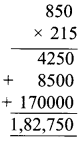Thus, 1,82,750 toffees are there in 215 packets.

(b) A can of oil contains 15 litres of oil. How much oil is there in 240 such cans?
1 can contains oil = 15 litres
240 can contains oil =15Thus, 3,600 litres of oil is present in 240 cans.

(c) There are 238 beads in a necklace. Find the total number of beads in 167 such necklaces.
No. of beads in 1 necklace = 238
No. of beads in 167 necklaces = 238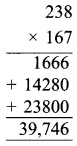Thus, number of beads in 167 necklaces is 39,746.(d) One dozen bananas cost ₹ 36. What is the cost of 720 dozen bananas?
1 dozen bananas cost = ₹ 36
720 dozen bananas cost = ₹ 36Thus, the cost of 720 dozen bananas is 25,920.

(e) There are 1,000 pages in a book. How many pages are there in 75 such books?
No. of pages in 1 book = 1000
No. of pages in 75 books = 1000 × 75
= 75,000
Thus, number of pages in 75 books is 75,000.

### DAV Class 4 Maths Chapter 3 Value Based Questions

Question 1.
If the cost of one fan is ₹ 895, how much money was spent on the fans?
Cost of one fan = ₹ 895
Cost of 35 fans = ₹ 895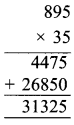Question 2.
In what other ways can you help a charitable hospital?
We can help a charitable hospital
(a) by collecting money.
(b) by donating bed or furnitures.

Word Problem

E.g. Rama saves ₹ 420 every month. How much money can be save
(i) in 12 months (ii) in 3 years?
Money saved by Rama in one month = ₹ 420
Money saved by Rama in 12 months = ₹ 420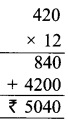Rama saves ₹ 5040 in 12 months.

(ii) one year =12 months
Money saved in one year = ₹ 5040
Money saved in 3 years = ₹ 5040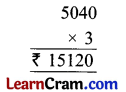In three years she can save ₹ 415,120.

Question 1.
Find the product orally.
(a) 572 × 0 = _____
0

(b) 150 × 1 = _____
150

(c) _____ × 1 = 260
260(d) 2,532 × _____ = 0
0

(e) 1,309 × 10 = _____
13,090

(f) 2,731 × 100 = _____
2,73,100

Question 2.
Fill in the blanks:
(i) (82×72) × 96 = 82 (_____ × 96)
72

(ii) 138 × (420 × 641) = (_____ × 420) × 641
138

(iii) _____ × (82 × 62) = (26 × _____) × 62
26, 82

(iv) 231 × _____ = _____ × 360
360; 231

(v) 102 × _____ = _____ × 326
326; 102

Question 3.
Multiply.
(i) 372 × 142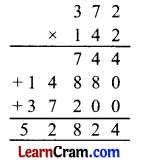(ii) 2312 × 131(iii) 246 × 30(iv) 1441 × 200Question 4.
Fill in the boxes.